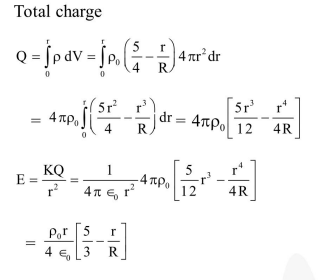# Let there be a spherically symmetric charge distribution with charge density varying as

Question:

Let there be a spherically symmetric charge distribution with charge density varying as $\rho(r)=\rho_{0}\left(\frac{5}{4}-\frac{r}{R}\right)$ upto $r=R$, and $\rho(r)=0$ for $r>R$, where $r$ is the distance from the origin. The electric field at a distance $r(r 1.$\frac{\rho_{0} \mathrm{r}}{3 \varepsilon_{0}}\left(\frac{5}{4}-\frac{\mathrm{r}}{\mathrm{R}}\right)$2.$\frac{4 \pi \rho_{0} r}{3 \varepsilon_{0}}\left(\frac{5}{3}-\frac{r}{R}\right)$3.$\frac{\rho_{0} r}{4 \varepsilon_{0}}\left(\frac{5}{3}-\frac{r}{R}\right)$4.$\frac{4 \rho_{0} r}{3 \varepsilon_{0}}\left(\frac{5}{4}-\frac{r}{R}\right)\$

Correct Option: , 3

Solution: